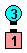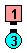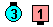Definitions of Square Dance Calls and Concepts

Index -->  Plus  |  A1  |  A2  |  C1  |  C2  |  C3A  |  C3B  |  C4  |  NOL  |
Definitions (Text Only) -->  Plus  |  A1  |  A2  |  C1  |  C2  |  C3A  |  C3B  |  C4  |  NOL  |
 Find call:

Single Circle To A Wave [Plus]
(author unknown)

From Facing Dancers.

Join hands (in a group of 2), Circle Left 1/2 way, drop hands and Step to a Right-Hand Mini-Wave.

Ends in a Right-Hand Mini-Wave.Single Circle To A Wave\$B\$NA0(B Single Circle 1/2\$B\$N8e(B Step To A Wave\$B\$N8e(B (\$B=*\$o\$j(B)

\$BCm
• Since the last part of Single Circle To A Wave is a Step To A Wave, nobody can Roll.
• \$B1~MQ(B:

• Single Circle fraction To A Wave:
Single Circle the given fraction then Step To A Wave. Fraction is usually 3/4 or 1/4.
• Single Circle fraction (without the words 'To A Wave'):
Single Circle the given fraction and remain facing each other.

Circle (fraction) To A Wave [C2] (Lee Kopman 1976): From Facing Couples. Circle Left 1/4 (or the given fraction); Beaus Walk as Belles Dodge. Ends in a R-H Mini-Wave Box.

Circle (fraction) To A Two-Faced Line [C4]: From Facing Couples. Circle Left 1/2 (or the given fraction); Veer Left. Ends in a R-H Two-Faced Line. Same as As Couples Single Circle To A Wave.Choreography for Single Circle To A WaveComments? Questions? Suggestions?